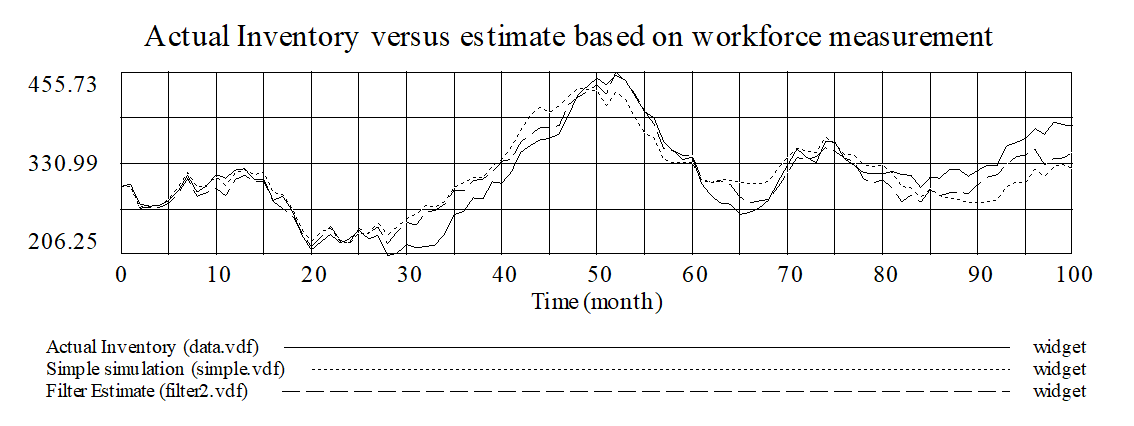# Vensim Help

 Simulating Incomplete Models

Simulating a model requires that equations be written for each variable.  Often, when developing a model, it is helpful to begin simulation experiments before a model has been completed.  Vensim provides a capability to do this using the Model>Partial Simulation… command.  This command simulates variables for which equations have been written while ignoring other variables.  Since some variables which have equations are likely to use other variables that do not have equations, you can specify a placeholder value for variables that do not yet have equations.

For example, suppose that you are developing a model of market growth with technological obsolescence as in:Here the inner positive feedback loop is fairly well developed, while the outer one is still pretty abstract and needs more detail to be added.  We might, nonetheless, want to simulate the positive loop before continuing with the rest of the model.  Suppose that we have the equations:

FRACTION REV SALES = 0.15

~ Dmnl ~|

indicated sales force = revenue * FRACTION REV SALES / WAGE RATE

~ Person ~|

PRICE = 100

~ \$/Widget ~|

product sales = sales force * sales force effectiveness

~ Widget/Year ~|

revenue = product sales * PRICE

~ \$/Month ~|

 sales force = INTEG((indicated sales force - sales force ) /